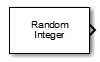Random Integer Generator

Generate integers randomly distributed in specified range

• Library:
• Communications Toolbox / Comm Sources / Random Data Sources

•Description

The Random Integer Generator block generates uniformly distributed random integers in the range [0, M-1], where M is specified by the Set size parameter. Use this block to generate random binary-valued or integer-valued data.

To ensure that the model uses different initial seeds, set the Simulate using parameter to Interpreted execution, and run the simulation in Normal or Accelerator mode. For more information, see Limitations.

Ports

Output

expand all

Random integer output, returned as a scalar, vector, or matrix. This port is unnamed on the block. The data type is set using the Output data type parameter.

The number of rows in the output data equals the value of the Samples per frame parameter and corresponds to the number of samples in one frame. The number of columns in the output data equals the number of elements in the Set size parameter and corresponds to the number of channels.

Parameters

expand all

Set size,M, specified as a positive integer or row vector of positive integers. The block generates integers in the range [0, (M – 1)]. The number of elements in Set size corresponds to the number of independent channels output from the block.

• If Set size is a scalar, then all output random variables are independent and identically distributed (i.i.d.).

• If Set size is a vector, then the length of the vector determines the number of output channels. The channels can have differing output ranges.

Source of the initial seed for the random number generator, specified as either:

• Auto –– the block uses the global random number stream

• Parameter –– the block sets the random number generator seed to Initial seed

Initial seed value for the random number generator, specified as a nonnegative integer. If the Initial seed parameter is a constant, then the resulting sequence is repeatable.

Dependencies

To enable this parameter, set the Source of initial seed parameter to Parameter.

Positive scalars specify the time in seconds between each sample of the output signal. If you set the Sample time to -1, the output signal inherits the sample time from downstream. For information on the relationship between the Sample time and Samples per frame parameters, see Sample Timing.

Samples per frame, specified as a positive integer indicating the number of samples per frame in one channel of the output data. For information on the relationship between Sample time and Samples per frame, see Sample Timing.

Output data type, specified as double, single, uint8, uint16, uint32, or boolean. If this parameter is set to boolean, you must set the Set size parameter to 2.

Type of simulation to run, specified as:

• Code generation –– Simulate the model using generated C code. The first time you run a simulation, Simulink® generates C code for the block. The C code is reused for subsequent simulations as long as the model does not change. This option requires additional startup time.

• Interpreted execution –– Simulate the model using the MATLAB® interpreter. This option shortens startup time. In Interpreted execution mode, you can debug the source code of the block.

Block Characteristics

 Data Types Boolean | double | integer | single Multidimensional Signals no Variable-Size Signals no

expand all

Compatibility Considerations

expand all

Behavior changed in R2020a